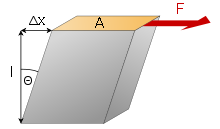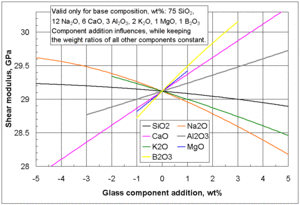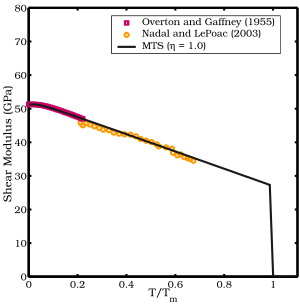# Shear modulus

﻿
Shear modulus
 Shear modulus SI symbol: G SI unit: gigapascal Derivations from other quantities: G = τ / γShear strain

In materials science, shear modulus or modulus of rigidity, denoted by G, or sometimes S or μ, is defined as the ratio of shear stress to the shear strain:$G \ \stackrel{\mathrm{def}}{=}\ \frac {\tau_{xy}} {\gamma_{xy}} = \frac{F/A}{\Delta x/l} = \frac{F l}{A \Delta x}$

where$\tau_{xy} = F/A \,$ = shear stress;
F is the force which acts
A is the area on which the force acts
in engineering,$\gamma_{xy} = \Delta x/l = \tan \theta \,$ = shear strain. Elsewhere, γxy = θ
Δx is the transverse displacement
l is the initial length

Shear modulus is usually expressed in gigapascals (GPa) or in thousands of pounds per square inch (kpsi).

The shear modulus is always positive.

## Explanation

Material Typical values for
shear modulus (GPa)
(at room temperature)
Diamond 478.
Steel 79.3
Copper 44.7
Titanium 41.4
Glass 26.2
Aluminium 25.5
Polyethylene 0.117
Rubber 0.0006

The shear modulus is one of several quantities for measuring the stiffness of materials. All of them arise in the generalized Hooke's law:

• Young's modulus describes the material's response to linear strain (like pulling on the ends of a wire),
• the bulk modulus describes the material's response to uniform pressure, and
• the shear modulus describes the material's response to shearing strains.

The shear modulus is concerned with the deformation of a solid when it experiences a force parallel to one of its surfaces while its opposite face experiences an opposing force (such as friction). In the case of an object that's shaped like a rectangular prism, it will deform into a parallelepiped. Anisotropic materials such as wood and paper exhibit differing material response to stress or strain when tested in different directions. In this case, when the deformation is small enough so that the deformation is linear, the elastic moduli, including the shear modulus, will then be a tensor, rather than a single scalar value.

## WavesInfluences of selected glass component additions on the shear modulus of a specific base glass.

In homogeneous and isotropic solids, there are two kinds of waves, pressure waves and shear waves. The velocity of a shear wave, (vs) is controlled by the shear modulus,$v_s = \sqrt{\frac {G} {\rho} }$

where

G is the shear modulus
ρ is the solid's density.

## Shear modulus of metalsShear modulus of copper as a function of temperature. The experimental data are shown with colored symbols.

The shear modulus of metals measures the resistance to glide over atomic planes in crystals of the metal. In polycrystalline metals there are also grain boundary factors that have to be considered. In metal alloys, the shear modulus is observed to be higher than in pure metals due to the presence of additional sources of resistance to glide.

The shear modulus of metals is usually observed to decrease with increasing temperature. At high pressures, the shear modulus also appears to increase with the applied pressure. Correlations between the melting temperature, vacancy formation energy, and the shear modulus have been observed in many metals.

Several models exist that attempt to predict the shear modulus of metals (and possibly that of alloys). Shear modulus models that have been used in plastic flow computations include:

1. the MTS shear modulus model developed by and used in conjunction with the Mechanical Threshold Stress (MTS) plastic flow stress model.
2. the Steinberg-Cochran-Guinan (SCG) shear modulus model developed by and used in conjunction with the Steinberg-Cochran-Guinan-Lund (SCGL) flow stress model.
3. the Nadal and LePoac (NP) shear modulus model that uses Lindemann theory to determine the temperature dependence and the SCG model for pressure dependence of the shear modulus.

### MTS shear modulus model

The MTS shear modulus model has the form:$\mu(T) = \mu_0 - \frac{D}{\exp(T_0/T) - 1}$

where µ0 is the shear modulus at 0 K, and D and T0 are material constants.

### SCG shear modulus model

The Steinberg-Cochran-Guinan (SCG) shear modulus model is pressure dependent and has the form$\mu(p,T) = \mu_0 + \frac{\partial \mu}{\partial p} \frac{p}{\eta^{1/3}} + \frac{\partial \mu}{\partial T}(T - 300) ; \quad \eta := \rho/\rho_0$

where, µ0 is the shear modulus at the reference state (T = 300 K, p = 0, η = 1), p is the pressure, and T is the temperature.

### NP shear modulus model

The Nadal-Le Poac (NP) shear modulus model is a modified version of the SCG model. The empirical temperature dependence of the shear modulus in the SCG model is replaced with an equation based on Lindemann melting theory. The NP shear modulus model has the form:$\mu(p,T) = \frac{1}{\mathcal{J}(\hat{T})} \left[ \left(\mu_0 + \frac{\partial \mu}{\partial p} \cfrac{p}{\eta^{1/3}} \right) (1 - \hat{T}) + \frac{\rho}{Cm}~k_b~T\right]; \quad C := \cfrac{(6\pi^2)^{2/3}}{3} f^2$

where$\mathcal{J}(\hat{T}) := 1 + \exp\left[-\cfrac{1+1/\zeta} {1+\zeta/(1-\hat{T})}\right] \quad \text{for} \quad \hat{T}:=\frac{T}{T_m}\in[0,1+\zeta],$

and µ0 is the shear modulus at 0 K and ambient pressure, ζ is a material parameter, kb is the Boltzmann constant, m is the atomic mass, and f is the Lindemann constant.

Wikimedia Foundation. 2010.

### Look at other dictionaries:

• Shear modulus — Shear modulus. См. Модуль сдвига (G). (Источник: «Металлы и сплавы. Справочник.» Под редакцией Ю.П. Солнцева; НПО Профессионал , НПО Мир и семья ; Санкт Петербург, 2003 г.) …   Словарь металлургических терминов

• shear modulus — Physics. a coefficient of elasticity of a substance, expressing the ratio between the force per unit area (shearing stress) that laterally deforms the substance and the shear (shearing strain) that is produced by this force. Also called modulus… …   Universalium

• shear modulus — šlyties modulis statusas T sritis Standartizacija ir metrologija apibrėžtis Liestinio įtempio ir santykinės šlyjamosios deformacijos dalmuo, t. y. G = τ/γ ; čia τ – liestinis įtempis, γ – santykinė šlyjamoji deformacija. atitikmenys: angl.… …   Penkiakalbis aiškinamasis metrologijos terminų žodynas

• shear modulus — šlyties modulis statusas T sritis fizika atitikmenys: angl. modulus of rigidity; shear modulus vok. Gleitmodul, m; Schermodul, m; Schubmodul, m rus. модуль сдвига, m pranc. module de cisaillement, m; module de rigidité, m; module d’élasticité au… …   Fizikos terminų žodynas

• shear modulus — noun : the ratio of the shearing stress in a body to the corresponding shearing strain * * * Physics. a coefficient of elasticity of a substance, expressing the ratio between the force per unit area (shearing stress) that laterally deforms the… …   Useful english dictionary

• shear modulus — /ˈʃɪə mɒdʒələs/ (say shear mojuhluhs) noun → modulus of rigidity …   Australian English dictionary

• shear modulus — the ratio of shear stress divided by the corresponding shear strain in a linear elastic material …   Mechanics glossary

• Shear stress — SI symbol: τ SI unit: pascal Derivations from other quantities: τ = F / A …   Wikipedia

• Shear strain — is a strain that acts parallel to the face of a material that it is acting on. Normal strain acts perpendicular to the face of that it is acting on. There are two ways to interpret shear strain: the average shear strain and the engineering shear… …   Wikipedia

• Shear strength — in engineering is a term used to describe the strength of a material or component against the type of yield or structural failure where the material or component fails in shear. In structural and mechanical engineering the shear strength of a… …   Wikipedia